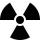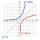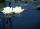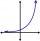Half life

Determine the half life of bismuth, when bismuth weight from the original weight of 32 g was only 2 grams in 242 minutes.

Result

t =  60.5 min

Solution:Leave us a comment of example and its solution (i.e. if it is still somewhat unclear...):Be the first to comment!To solve this example are needed these knowledge from mathematics:

Do you have a linear equation or system of equations and looking for its solution? Or do you have quadratic equation?

Next similar examples:After 548 hours decreases the activity of a radioactive substance to 1/9 of the initial value. What is the half-life of the substance?
2. LogarithmDetermine the number whose decimal logarithm is -3.8.
3. CoordinateDetermine missing coordinate of the point M [x, 120] of the graph of the function f bv rule: y = 5x
4. Theorem proveWe want to prove the sentence: If the natural number n is divisible by six, then n is divisible by three. From what assumption we started?
5. DemographicsThe population grew in the city in 10 years from 42000 to 54500. What is the average annual percentage increase of population?
6. Logif ?, what is b?
7. SequenceCalculate what member of the sequence specified by ? has value 86.
8. Insert into GPBetween numbers 5 and 640 insert as many numbers to form geometric progression so sum of the numbers you entered will be 630. How many numbers you must insert?
9. The city 3The city has 22,000 residents. How long it is expected to have 25,000 residents if the average annual population growth is 1.4%?
10. Six termsFind the first six terms of the sequence a1 = -3, an = 2 * an-1
11. PopulationWhat is the population of the city with 3% annual growth, if in 10 years the city will have 60,000 residents?
12. Exponential equationIn the set R solve the equation: ?
13. Geometric progression 2There is geometric sequence with a1=5.7 and quotient q=-2.5. Calculate a17.
14. Intercept with axisF(x)=log(x+4)-2, what is the x intercept
15. Water liliesWater lilies are growing on the pond and their number is doubled every day. The whole layer is covered in 12 days. How many days will it cover 8 layers?
16. PopulationThe town has 65,000 inhabitants. 40 years ago there were 157,000. How many people will live in town in 10 years if the average rate in population is as in previous years?
17. Powers 32 to the power of n divided by 4 to the power of -3 equal 4. What is the vaule of n?